BRAIN TEASERS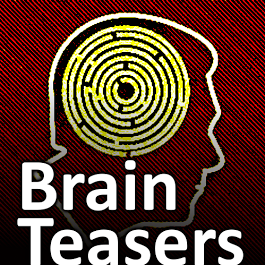# Monthly Archive - March 2023

brain teasers, puzzles, riddles, mathematical problems, mastermind, cinemania... These are the tasks listed 1 to 10.

## Calculate the number 6633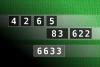NUMBERMANIA: Calculate the number 6633 using numbers [4, 2, 6, 5, 83, 622] and basic arithmetic operations (+, -, *, /). Each of the numbers can be used only once.
#brainteasers #math #numbermania

## MAGIC SQUARE: Calculate A*B*C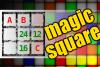The aim is to place the some numbers from the list (4, 7, 8, 12, 15, 16, 21, 24, 25, 41, 93, 97) into the empty squares and squares marked with A, B an C. Sum of each row and column should be equal. All the numbers of the magic square must be different. Find values for A, B, and C. Solution is A*B*C.
#brainteasers #math #magicsquare

## Find number abc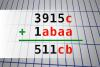If 3915c + 1abaa = 511cb find number abc. Multiple solutions may exist.
#brainteasers #math### Can opener

What do you call a broken can opener?

A can’t opener.

Posted by Melusedek on Reddit on Nov 14. 2013

Jokes of the day - Daily updated jokes. New jokes every day.

## Find the right combination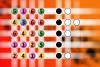The computer chose a secret code (sequence of 4 digits from 1 to 6). Your goal is to find that code. Black circles indicate the number of hits on the right spot. White circles indicate the number of hits on the wrong spot.
#brainteasers #mastermind

## Calculate the number 2229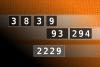NUMBERMANIA: Calculate the number 2229 using numbers [3, 8, 3, 9, 93, 294] and basic arithmetic operations (+, -, *, /). Each of the numbers can be used only once.
#brainteasers #math #numbermania

## Find number abc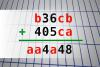If b36cb + 405ca = aa4a48 find number abc. Multiple solutions may exist.
#brainteasers #math

## What a winning combination?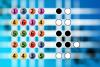The computer chose a secret code (sequence of 4 digits from 1 to 6). Your goal is to find that code. Black circles indicate the number of hits on the right spot. White circles indicate the number of hits on the wrong spot.
#brainteasers #mastermind

## Calculate the number 2193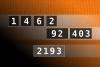NUMBERMANIA: Calculate the number 2193 using numbers [1, 4, 6, 2, 92, 403] and basic arithmetic operations (+, -, *, /). Each of the numbers can be used only once.
#brainteasers #math #numbermania

## MAGIC SQUARE: Calculate A-B+C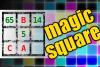The aim is to place the some numbers from the list (2, 3, 5, 6, 11, 14, 36, 65, 66, 70, 74, 78) into the empty squares and squares marked with A, B an C. Sum of each row and column should be equal. All the numbers of the magic square must be different. Find values for A, B, and C. Solution is A-B+C.
#brainteasers #math #magicsquare

## Find number abc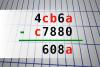If 4cb6a - c7880 = 608a find number abc. Multiple solutions may exist.
 1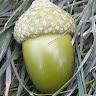H Tav 5861 2c. raj. 5777 3Nasrin 24 T 5731 4Fazil Hashim 5718 5Thinh Ddh 5557 6Alfa Omega 4681 7Djordje Timotijevic 4549 8Mita Kojd 4525 9Jakubovski Vladimir 4443 10Chandu Rajyaguru 4419